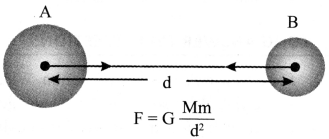## JAC Class 9 Science Notes Chapter 10 Gravitation

### JAC Board Class 9th Science Chapter 10 Notes Gravitation

→ Gravitation:
The universal law of gravitation’ states that any two bodies having mass, attract each other with a force which is directly proportional to the product of their masses and inversely proportional to the square of the distance between them. The force acts along the line joining the centres of the objects.Here, G is universal gravitational constant with a value of 6.673 × 10-11 N m2 kg2. The value of G was found out by Henry Cavendish.

Note:

• If mass of an object is large, force will be more. If mass of an object is small, force will be less.
• If distance between two objects is more, force exerted will be less and vice versa.→ Importance of Gravitational Force

• It binds us to the earth.
• Moon revolves around the earth due to gravitational force. Planets revolve around the sun due to gravitational force.
• Tides in oceans are caused due to gravitational force of the moon on earth.

→ Centripetal acceleration of the moon:
If the moon is revolving with speed v in a circular orbit of radius r, then the acceleration acting on it along the radius and towards the centre of its orbit is
ac = $$\frac{\mathrm{v}^{2}}{\mathrm{r}}$$

→ Free fall: The earth attracts objects towards it due to gravitational force. When an object moves under the influence of gravitational force alone, it is said to be in a ‘free fall’.

→ Acceleration due to gravity: The acceleration produced in the bodies due to earth’s gravity is called acceleration due to gravity. Its value on the earth’s surface is 9.8 m/s2.

→ Relations between g and G: If M is the mass of the earth and R is its radius, the acceleration due to gravity at the surface of the earth is given by
g = $$\frac{\mathrm{GM}}{(\mathrm{R})^{2}}$$

The value of g depends on:

• shape of the earth
• height above the earth
• depth inside the earth
• latitude on the earth

→ Mass of the earth:
It is given by
M = $$\frac{g^{2}}{G}$$

→ Centre of mass:
The centre of mass of a body may be defined as the point at which whole mass of the body may be assumed to be concentrated.

→ Centre of gravity:
The centre of gravity of a body is a point at which the resultant of all the parallel forces due to gravity, experienced by various particles of the body, acts or at which whole weight of the body acts.

→ Equations of motion for freely falling bodies: The three equations of motion are:
v = u + at ……..(ii)
s = ut + $$\frac{1}{2}$$ at2 …..(ii)
v2 – u2 = 2as
= g = 9.8 ms-2

→ Projectile: Any object thrown into space with some initial velocity and which thereafter moves under the influence of gravity alone is called a ‘projectile’. The path of a projectile is a parabola. Its horizontal range is maximum when the angle of projection is 45°.→ Mass: Mass of an object is the measure of its inertia. It is the amount of matter present in it. It remains the same everywhere in the universe.

→ Weight: The force with which an object is attracted towards the centre of the earth is known as the weight of that object. Its SI unit is newton.
W = m × g
where W = weight of the object, m = mass of the object, and g = acceleration due to gravity.
The weight of an object can change from one place to the other and from one planet to the other.

→ Thrust: It is the net force applied in a particular direction. Its SI unit is N.
Pressure = $$\frac{\text { Thrust }}{\text { Area }}$$
The SI unit of density is kg/m3.

→ Relative density: It is the ratio of the density of a substance to the density of water at 4°C.
Density of substance Density of water at 4°C
Since relative density is a pure ratio, it has no units.

→ Pressure in fluids:
All liquids and gases are fluids as they can flow. The pressure exerted by a fluid is transmitted in all directions.

→ Buoyancy:
The upward force exerted by water (fluid) on the body immersed in it is known as upthrust or buoyancy. The magnitude of the buoyant force depends of the density of the fluid.

→ Archimedes’ principle: It states that when a body is immersed fully or partially in a fluid, it experiences an upward force that is equal to the weight of the fluid displaced by it.
It has following applications:

• Used in designing ships and submarines.
• In lactometers to find purity of milk.
• In hydrometers to determine the density of a liquid.→ Density: The density of a substance is its mass per unit volume.
Density = $$\frac{\text { Mass }}{\text { Volume }}$$
The SI unit of density is kg/m3.

→ Relative density: It is the ratio of the density of a substance to the density of water at 4°C.
Density of substance Density of water at 4°C
Since relative density is a pure ratio, it has no units.